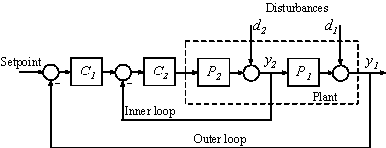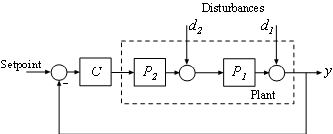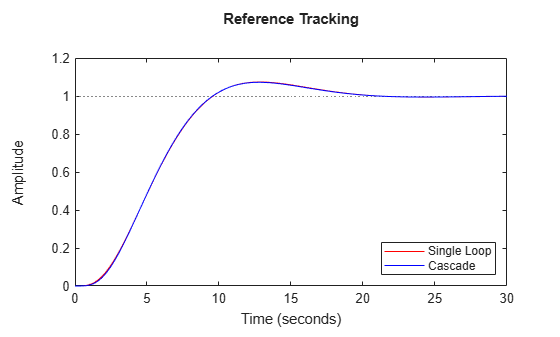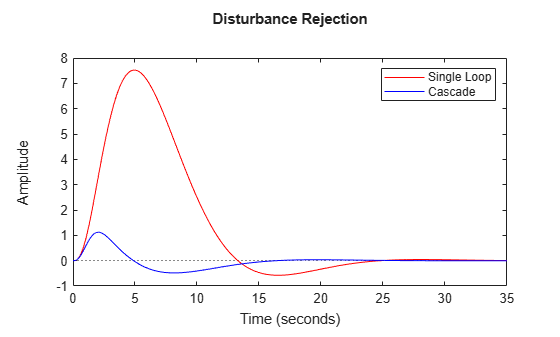Documentation

## Designing Cascade Control System with PI Controllers

This example shows how to design a cascade control loop with two PI controllers using the pidtune command.

Cascade control is mainly used to achieve fast rejection of disturbance before it propagates to the other parts of the plant. The simplest cascade control system involves two control loops (inner and outer) as shown in the block diagram below.Controller C1 in the outer loop is the primary controller that regulates the primary controlled variable y1 by setting the set-point of the inner loop. Controller C2 in the inner loop is the secondary controller that rejects disturbance d2 locally before it propagates to P1. For a cascade control system to function properly, the inner loop must respond much faster than the outer loop.

In this example, you will design a single loop control system with a PI controller and a cascade control system with two PI controllers. The responses of the two control systems are compared for both reference tracking and disturbance rejection.

### Plant

In this example, the inner loop plant P2 is

`$P2\left(s\right)=\frac{3}{s+2}$`

The outer loop plant P1 is

`$P1\left(s\right)=\frac{10}{\left(s+1{\right)}^{3}}$`

```P2 = zpk([],-2,3); P1 = zpk([],[-1 -1 -1],10);```

### Designing a Single Loop Control System with a PI Controller

Use pidtune command to design a PI controller in standard form for the whole plant model P = P1 * P2.The desired open loop bandwidth is 0.2 rad/s, which roughly corresponds to the response time of 10 seconds.

```% The plant model is P = P1*P2 P = P1*P2; % Use a PID or PIDSTD object to define the desired controller structure C = pidstd(1,1); % Tune PI controller for target bandwidth is 0.2 rad/s C = pidtune(P,C,0.2); C```
```C = 1 1 Kp * (1 + ---- * ---) Ti s with Kp = 0.0119, Ti = 0.849 Continuous-time PI controller in standard form ```

### Designing a Cascade Control System with Two PI Controllers

The best practice is to design the inner loop controller C2 first and then design the outer loop controller C1 with the inner loop closed. In this example, the inner loop bandwidth is selected as 2 rad/s, which is ten times higher than the desired outer loop bandwidth. In order to have an effective cascade control system, it is essential that the inner loop responds much faster than the outer loop.

Tune inner-loop controller C2 with open-loop bandwidth at 2 rad/s.

```C2 = pidtune(P2,pidstd(1,1),2); C2```
```C2 = 1 1 Kp * (1 + ---- * ---) Ti s with Kp = 0.244, Ti = 0.134 Continuous-time PI controller in standard form ```

Tune outer-loop controller C1 with the same bandwidth as the single loop system.

```% Inner loop system when the control loop is closed first clsys = feedback(P2*C2,1); % Plant seen by the outer loop controller C1 is clsys*P1 C1 = pidtune(clsys*P1,pidstd(1,1),0.2); C1```
```C1 = 1 1 Kp * (1 + ---- * ---) Ti s with Kp = 0.015, Ti = 0.716 Continuous-time PI controller in standard form ```

### Performance Comparison

First, plot the step reference tracking responses for both control systems.

```% single loop system for reference tracking sys1 = feedback(P*C,1); sys1.Name = 'Single Loop'; % cascade system for reference tracking sys2 = feedback(clsys*P1*C1,1); sys2.Name = 'Cascade'; % plot step response figure; step(sys1,'r',sys2,'b') legend('show','location','southeast') title('Reference Tracking')```Secondly, plot the step disturbance rejection responses of d2 for both control systems.

```% single loop system for rejecting d2 sysd1 = feedback(P1,P2*C); sysd1.Name = 'Single Loop'; % cascade system for rejecting d2 sysd2 = P1/(1+P2*C2+P2*P1*C1*C2); sysd2.Name = 'Cascade'; % plot step response figure; step(sysd1,'r',sysd2,'b') legend('show') title('Disturbance Rejection')```From the two response plots you can conclude that the cascade control system performs much better in rejecting disturbance d2 while the set-point tracking performances are almost identical.